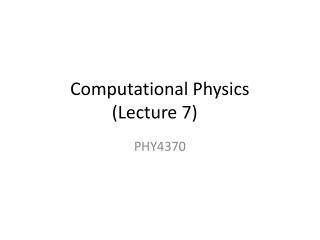DownloadDownload PresentationComputational Physics (Lecture 7)

# Computational Physics (Lecture 7)

Download Presentation## Computational Physics (Lecture 7)

- - - - - - - - - - - - - - - - - - - - - - - - - - - E N D - - - - - - - - - - - - - - - - - - - - - - - - - - -
##### Presentation Transcript

1. Quadratic form • A quadratic form is simply a scalar, quadratic function of a vector with the form • A is the matrix, x and b are vectors, c is a scalar constant. If A is symmetric and positive definite, f(x) is minimized by the solution to Ax=b.

2. Sample Problem: • c= 0

3. The gradient • ,…,=-b. • If A is symmetric, the equation becomes: Ax-b.

4. Revisit of Steepest descent • We start from an arbitrary starting point: x(0), • Slide down to the bottom of the paraboloid. • When we take the step, we choose the direction in which f decreases most quickly. • -f’(x(i))=b-Ax(i). • Error: e(i) = x(i)-x => how far from the solution. • Residual: r(i) = b-Ax(i) => how far from the correct value of b.

5. Suppose we start from (-2, -2). Along the steepest descent, will fall somewhere on the solid line. • X(1) = x(0)+αr(0) • How big the step we should take? • Line search • Choose α to minimize f. df(x(1))/dα=0, • So, f’(x(1))Tr(0)=0 • So α is chosen to make r(0) and f’(x(1)) orthogonal!

6. Determine α • F(x(1))= -r(1) • r(1)Tr(0) = 0 • (b-Ax(1))Tr(0) = 0 • (b-A(x(0)+αr(0)))Tr(0) = 0 • (b-Ax(0))Tr(0) = α(Ar(0))Tr(0) • r(0) Tr(0)=αr(0)T(Ar(0)) • α =(r(0) Tr(0))/(r(0)TAr(0))

7. General method of Steepest Descent

8. Conjugate directions • SD often takes steps in the same direction! • If we take n orthogonal steps, each step has the correct length, afte n steps, we are done！ • In general, for each step, we have • x(i+1)=x(i)+α(i)d(i) • e(i+1) should be orthogonal to d(i) • d(i)Te(i+1) = 0 • d(i)T (e(i)+ α(i)d(i))=0 • α(i)=-d(i) Te(i)/(d(i) Td(i))

9. Useless! We don’t know e(i). • Instead, we make d(i) and d(j) A-orthogonal, or conjugate. • d(i)TAd(j) = 0

10. New requirement: • e(i+1) is A-orthogonal to d(i) • Like SD, we need to find the minimum along d(i)

11. This time, evaluate the α(i) again,

12. Conjugate Gradients • Just the Conjugate directions method where the search directions are constructed by the conjugation of the residuals. • ui=r(i)

13. General Method

14. Sample code for SD

15. Multi variable Newton method • f(x) = 0, (f = ( f1, f2, . . . , fn) and x = (x1, x2, . . . , xn).) • Taylor expansion at Xr: • f(xr) = f(x) + x ·∇f(x) + O(x2) ≃0, • AΔx = b, • Aij= ∂ fi(x)/∂xj • Bi=-fi • For Newtonian method: • Xk+1 = xk+ Δxk • Secant Method • Aij= (fi(x + hj<xj>) − fi(x))/hj • hj≃δ0 xj • δ0 isthe tolerance of the floating point of data. • f1(x, y) = exp(x^2) lny − x^2 = 0 and f2(x, y) = exp(y2) lnx − y^2 = 0, around x = y = 1.5.

16. // An example of searching for the root via the secant method // for f_i[x_k] for i=1,2,...,n. import java.lang.*; public class Rootm { public static void main(String argv[]) { int n = 2; intni = 10; double del = 1e-6; double x[] = {1.5, 1.5}; secantm(ni, x, del); // Output the root obtained System.out.println("The root is at x = " + x + "; y = " + x); } // Method to carry out the multivariable secant search. public static void secantm(intni, double x[], double del) { int n = x.length; double h = 2e-5; int index[] = new int[n]; double a[][] = new double[n][n]; int k = 0; double dx = 0.1; while ((Math.abs(dx)>del) && (k<ni)) { double b[] = f(x); for (int i=0; i<n; ++i) { for (int j=0; j<n; ++j) { double hx = x[j]*h; x[j] += hx; double c[] = f(x); a[i][j] = (c[i]-b[i])/hx; } } dx = Math.sqrt(dx/n); k++; } if (k==n) System.out.println("Convergence not" + " found after " + ni + " iterations"); } public static double[] solve(double a[][], double b[], int index[]) {...} public static void gaussian(double a[][], int index[]) {...} // Method to provide function f_i[x_k]. public static double[] f(double x[]) { double fx[] = new double; fx = Math.exp(x*x)*Math.log(x) -x*x; fx = Math.exp(x)*Math.log(x)-x*x; return fx; } }

17. Extremes of a multi variable function, BFBG • f(x) = ∇g(x) = 0,